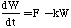## A Quick Glimpse at the Math

So far, we've represented this system in the form of a drawing and in the form of a STELLA model; one additional way to represent this system is in the form of a set of equations. In fact, this is what STELLA does behind the scenes. Here are the equations used to represent the first system - the one with negative feedback:

Faucet = 1.0 {l/sec} (1)

Drain = 0.1 * Water Tub {/sec *l} (2)

INIT Water Tub = 10 {l} (3)

Water Tub(t) = Water Tub(t-DT)+ (Faucet-Drain)*DT {l + l/sec * sec} (4)

Just to show that there is nothing too difficult about these equations, let's look at them a little more closely. Equations 1-3 are the starting conditions for the system; Eq. 1 specifies that the Faucet flow rate is constant at 1 liter/second; Eq. 2 specifies that the Drain flow rate is 0.1 multiplied by the number of liters in the water tub at any given time (t); Eq. 3 simply fixes the starting volume of water in tub to be 10 liters.

So at the very start, at time t=0, the value for the drain flow will be 1 liter/second, exactly the same as the faucet flow rate. The final equation is used by STELLA to keep track of how much water is in the tub throughout time. As the program runs, time changes from 0 to some specified value in time steps (DT) that are also specified by the user, so Water Tub(t) is the amount of water a particular time, Water Tub(t-DT) is the amount in the tub in the previous time step, and (Faucet-Drain)*DT is the difference in the inflow and outflow rates over the time step. You can see that the program is basically doing some accounting - adding material and subtracting material in small increments.

Another way of writing Eq. 4 is slightly more abstract, but familiar to mathematicians:where F represents the faucet flow rate while kW represents the drain flow rate. This is called a differential equation; it is discussed more fully in Appendix A, at the end of this chapter, where you can see what is involved in solving the equation without the use of a computer.